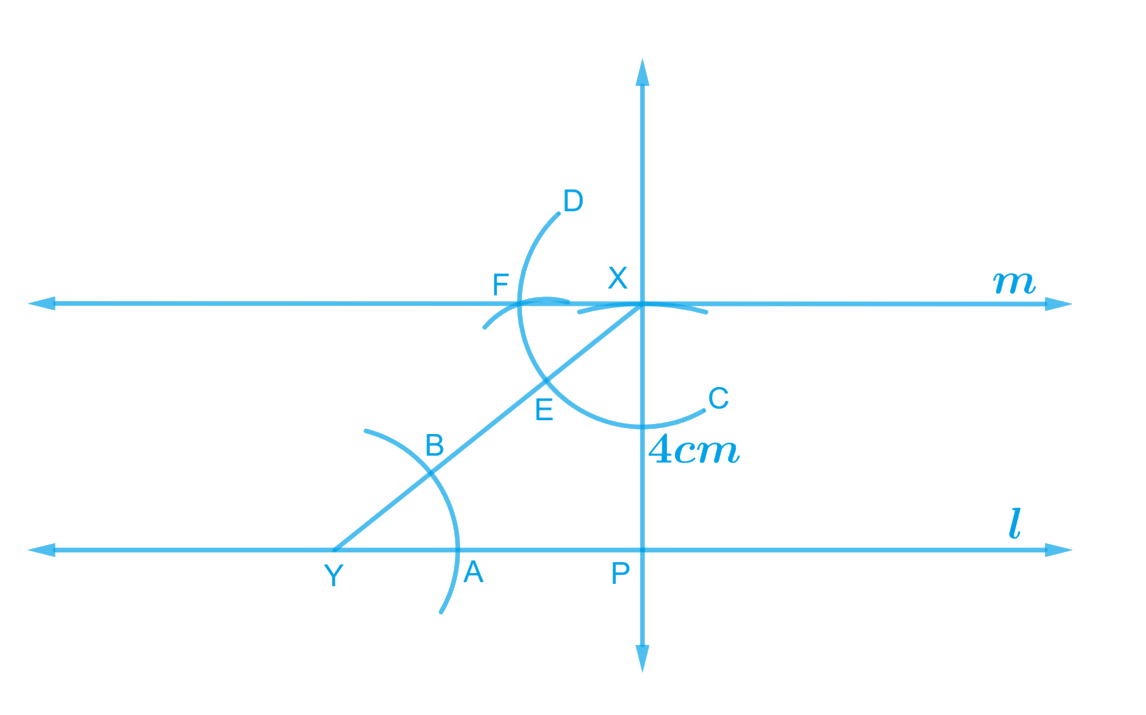# Ex.10.1 Q2 Practical Geometry Solution - NCERT Maths Class 7

Go back to  'Ex.10.1'

## Question

Draw a line $$l$$. Draw a perpendicular to $$l$$ at any point on $$l$$. On this perpendicular choose a point $$X$$, $$4 \rm\, cm$$ away from $$l$$. Through $$X$$, draw a line $$m$$ parallel to $$l$$.

Video Solution
Practical Geometry
Ex 10.1 | Question 2

## Text Solution

What is known?

A line $$l$$ and $$m$$ which are $$4\,\rm{cm}$$ apart.

To construct:

A perpendicular at any point on line $$l$$, and then draw a line $$m$$ parallel to $$l$$ through $$X$$ on the perpendicular which is $$4$$ cm from the line $$l$$.

Reasoning:

Draw a line $$l$$ and then a perpendicular to $$l$$ at any point on $$l$$. On this perpendicular choose a point $$X$$, $$4$$ cm away from $$l$$. Through $$X$$, draw a line $$m$$ parallel to $$l$$. Follow the steps given below.

Steps:Steps of construction

1. Draw a line $$l$$, take a point $$P$$ on it. Draw a perpendicular passing trough point $$P.$$
2. Now adjust the compass up-to the length of $$4$$ $$\rm cm.$$ Draw an arc to intersect this perpendicular at point $$X$$ choose any point $$Y$$ on line $$l$$, join $$X$$ to $$Y$$.
3. Taking $$Y$$ as centre and with a convenient radius, draw an arc intersecting $$l$$ at $$A$$ and $$XY$$ at $$B$$.
4. Taking $$X$$ as centre and with the same radius as above, draw an arc $$CD$$ cutting $$XY$$ at $$E$$Adjust the compass up-to to the length of $$AB$$.
5. Without changing the opening of compass and taking $$E$$ as the centre, draw an arc to intersect the previously drawn arc $$CD$$ at $$F$$.
6. Join the points $$X$$ and$$F$$ to draw a line $$\rm m.$$

Line $$\rm m$$ is the required line which is parallel to $$l$$.

Learn from the best math teachers and top your exams

• Live one on one classroom and doubt clearing
• Practice worksheets in and after class for conceptual clarity
• Personalized curriculum to keep up with school